##### The Japanese Bonsai specialist
Direct order Contact Help / Services Newsletter# Whetstone sharpener fine grain

Pierre d'aiguisage a eau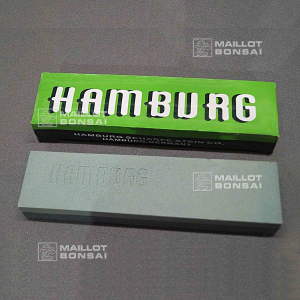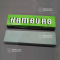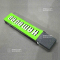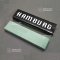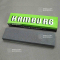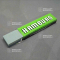ref. : 11884

11,50

Available quantity : 5Order

###### Description

Measurements: 200*50*25 mm. This is the solution for keeping tools sharp. The thin grain 120/320 is designed for sharpening scissors as well as jin and shari tools. Before sharpening the blades use our "kaneshin" eraser to clean off resin and sap or a solvent that doesn’t damage the whetstone. Soak the stone for at least 10 minutes in water and use the sloping side to sharpen scissor blades. Made in Germany.

#tools 4.5 #whetstone 3.9 #grain 3.5 #sharpening 3 #sharpener 2.9 #bonsai 2.6 #blades 2.6 #fine 2.4 #measurements 2.2 #solution 1.8

###### Technical description
 I would like to...

Formule
(( ROUND((CHAR_LENGTH(b.article_nom)-CHAR_LENGTH(REPLACE(b.article_nom, 'whetstone', '')))/LENGTH('whetstone')) + ROUND((CHAR_LENGTH(b.article_description)-CHAR_LENGTH(REPLACE(b.article_description, 'whetstone', '')))/LENGTH('whetstone')) ) * 3.9) + (( ROUND((CHAR_LENGTH(b.article_nom)-CHAR_LENGTH(REPLACE(b.article_nom, 'grain', '')))/LENGTH('grain')) + ROUND((CHAR_LENGTH(b.article_description)-CHAR_LENGTH(REPLACE(b.article_description, 'grain', '')))/LENGTH('grain')) ) * 3.5) + (( ROUND((CHAR_LENGTH(b.article_nom)-CHAR_LENGTH(REPLACE(b.article_nom, 'sharpening', '')))/LENGTH('sharpening')) + ROUND((CHAR_LENGTH(b.article_description)-CHAR_LENGTH(REPLACE(b.article_description, 'sharpening', '')))/LENGTH('sharpening')) ) * 3) + (( ROUND((CHAR_LENGTH(b.article_nom)-CHAR_LENGTH(REPLACE(b.article_nom, 'sharpener', '')))/LENGTH('sharpener')) + ROUND((CHAR_LENGTH(b.article_description)-CHAR_LENGTH(REPLACE(b.article_description, 'sharpener', '')))/LENGTH('sharpener')) ) * 2.9) + (( ROUND((CHAR_LENGTH(b.article_nom)-CHAR_LENGTH(REPLACE(b.article_nom, 'blades', '')))/LENGTH('blades')) + ROUND((CHAR_LENGTH(b.article_description)-CHAR_LENGTH(REPLACE(b.article_description, 'blades', '')))/LENGTH('blades')) ) * 2.6) + (( ROUND((CHAR_LENGTH(b.article_nom)-CHAR_LENGTH(REPLACE(b.article_nom, 'tools', '')))/LENGTH('tools')) + ROUND((CHAR_LENGTH(b.article_description)-CHAR_LENGTH(REPLACE(b.article_description, 'tools', '')))/LENGTH('tools')) ) * 2.5) + (( ROUND((CHAR_LENGTH(b.article_nom)-CHAR_LENGTH(REPLACE(b.article_nom, 'fine', '')))/LENGTH('fine')) + ROUND((CHAR_LENGTH(b.article_description)-CHAR_LENGTH(REPLACE(b.article_description, 'fine', '')))/LENGTH('fine')) ) * 2.4) + (( ROUND((CHAR_LENGTH(b.article_nom)-CHAR_LENGTH(REPLACE(b.article_nom, 'measurements', '')))/LENGTH('measurements')) + ROUND((CHAR_LENGTH(b.article_description)-CHAR_LENGTH(REPLACE(b.article_description, 'measurements', '')))/LENGTH('measurements')) ) * 2.2) + (( ROUND((CHAR_LENGTH(b.article_nom)-CHAR_LENGTH(REPLACE(b.article_nom, 'solution', '')))/LENGTH('solution')) + ROUND((CHAR_LENGTH(b.article_description)-CHAR_LENGTH(REPLACE(b.article_description, 'solution', '')))/LENGTH('solution')) ) * 1.8) + (( ROUND((CHAR_LENGTH(b.article_nom)-CHAR_LENGTH(REPLACE(b.article_nom, 'scissors', '')))/LENGTH('scissors')) + ROUND((CHAR_LENGTH(b.article_description)-CHAR_LENGTH(REPLACE(b.article_description, 'scissors', '')))/LENGTH('scissors')) ) * 1.8)

## Secure payment## Delivery

Our logistic partners :04 74 55 23 48
Pépinière MAILLOT-BONSAÏ
Le Bois Frazy
01990 RELEVANT - FRANCE
on appointment